# ormap and filter CS 5010 Program Design Paradigms

• Slides: 19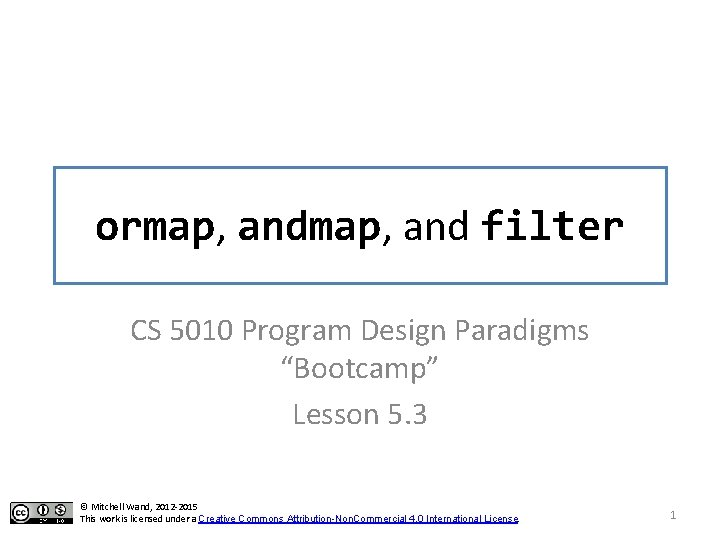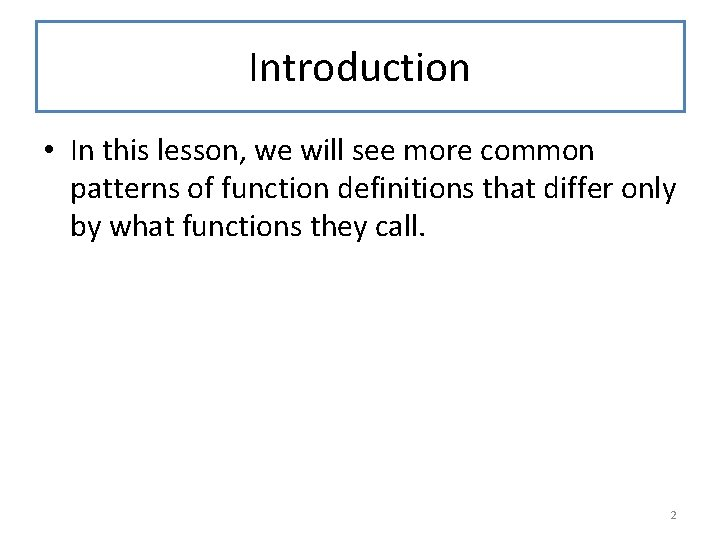Introduction • In this lesson, we will see more common patterns of function definitions that differ only by what functions they call. 2Learning Objectives • At the end of this lesson you should be able to: – recognize the ormap, and filter patterns – state the contracts for ormap, and filter , and use them appropriately. – combine these functions using higher-order function combination 3Let's look at find-dog again ; ; find-dog : List. Of. String -> Boolean ; ; RETURNS: true if "dog" is in the given list. ; ; STRATEGY: Use template for List. Of. String on los (define (find-dog los) (cond [(empty? los) false] [else (or (string=? (first los) "dog") (find-dog (rest los)))])) (check-equal? (find-dog (list "cat" "dog" "weasel")) true) (check-equal? (find-dog (list "cat" "elephant" "weasel")) false) 4Here's another function with a similar structure ; ; has-even? : List. Of. Integer -> Boolean ; ; RETURNS: true iff the given list contains ; ; an even number ; ; STRATEGY: Use List. Of. Integer on los (define (has-even? los) (cond [(empty? los) false] [else (or (even? (first los)) (has-even? (rest los)))])) 5Let's compare (define (find-dog los) (cond [(empty? los) false] [else (or (string=? (first los) "dog") (find-dog (rest los)))])) (define (has-even? los) (cond [(empty? los) false] [else (or (even? (first los) ) (has-even? (rest los)))])) 6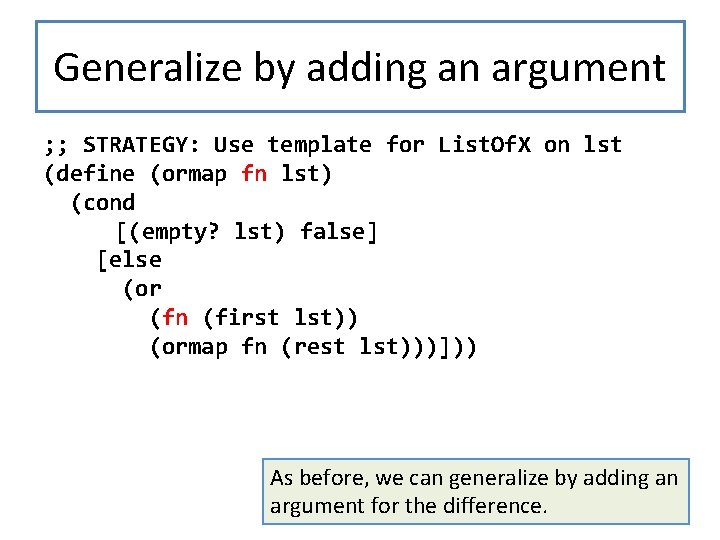Generalize by adding an argument ; ; STRATEGY: Use template for List. Of. X on lst (define (ormap fn lst) (cond [(empty? lst) false] [else (or (fn (first lst)) (ormap fn (rest lst)))])) As before, we can generalize by adding an argument for the difference. 7And re-create the originals Again as before, we recreate the originals using our generalized function. ; ; STRATEGY: Use HOF ormap on lst (define (find-dog lst) (ormap ; ; String -> Boolean (lambda (str) (string=? "dog" str)) lst))) ; ; STRATEGY: Use HOF ormap on lst (define (has-even? lst) (ormap even? lst)) If you're afraid of lambda, you can define is-dog? or use a local. But it's good to get comfortable with lambda– it's so useful that it was added to Java as of Java 8. 8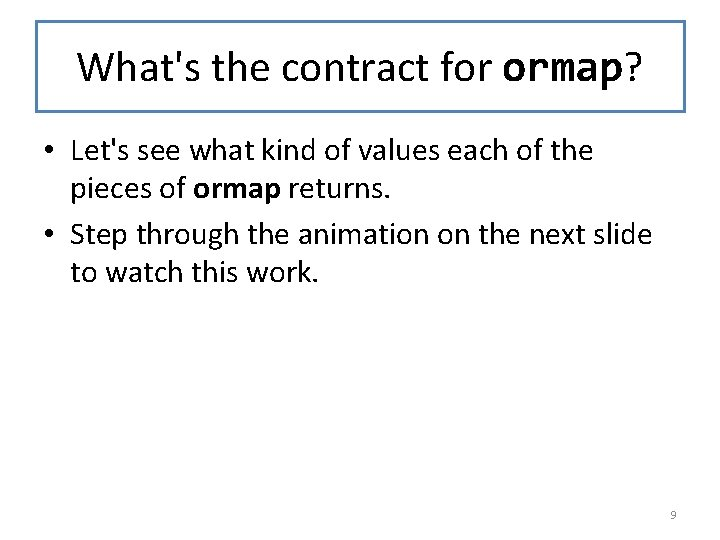What's the contract for ormap? • Let's see what kind of values each of the pieces of ormap returns. • Step through the animation on the next slide to watch this work. 9What's the contract? ormap : (X -> Bool) List. Of. X -> Bool fn must take an X, because (define (ormap fn lst) its argument Boolean is an X, and it must return a boolean, (cond because its return value is Both branches of the cond an argument to or. booleans, so ormap [(empty? lst) false] return must return a Boolean [else X List. Of. X (or Boolean (first lst)) (ormap fn (rest lst)))])) X -> Bool So fn must be a function from X's to Booleans, and lst must be a 10 List. Of. X. We write all this down in the contract.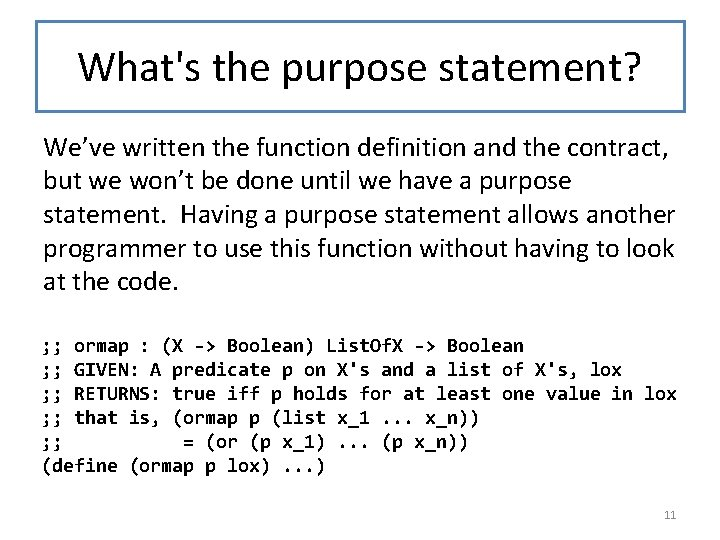What's the purpose statement? We’ve written the function definition and the contract, but we won’t be done until we have a purpose statement. Having a purpose statement allows another programmer to use this function without having to look at the code. ; ; ormap : (X -> Boolean) List. Of. X -> Boolean ; ; GIVEN: A predicate p on X's and a list of X's, lox ; ; RETURNS: true iff p holds for at least one value in lox ; ; that is, (ormap p (list x_1. . . x_n)) ; ; = (or (p x_1). . . (p x_n)) (define (ormap p lox). . . ) 11And of course we can do the same thing for and. (define (andmap fn lst) (cond [(empty? lst) true] [else (and (fn (first lst)) (andmap fn (rest lst)))])) 12Contract and Purpose Statement ; ; ; ; andmap : (X -> Bool) List. Of. X -> Bool GIVEN: A predicate p on X's and a list of X's, lox RETURNS: true iff p holds for every value in lox that is, (andmap p (list x_1. . . x_n)) = (and (p x_1). . . (p x_n)) The contract and purpose statement look very much like the ones for ormap. 13Another common pattern • Another common list-manipulation problem is to take a list and return a list of those values in the list that pass a certain test. • For example, here's a function that returns only the even values in a list of integers. 14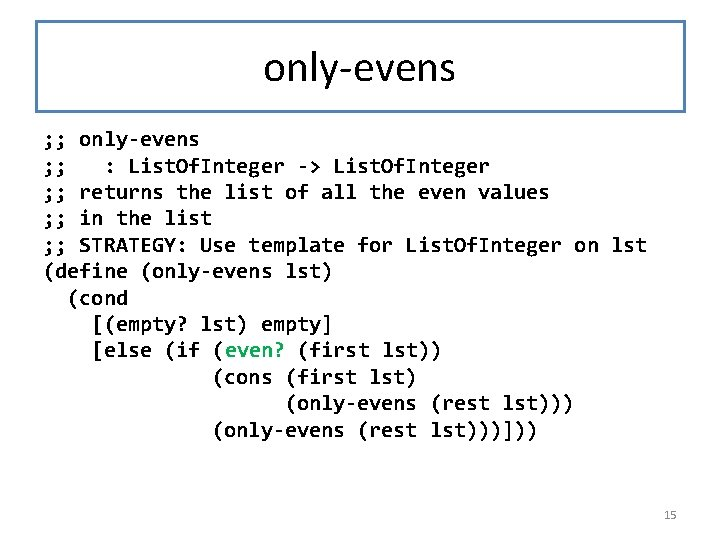only-evens ; ; : List. Of. Integer -> List. Of. Integer ; ; returns the list of all the even values ; ; in the list ; ; STRATEGY: Use template for List. Of. Integer on lst (define (only-evens lst) (cond [(empty? lst) empty] [else (if (even? (first lst)) (cons (first lst) (only-evens (rest lst)))])) 15Generalize: filter ; ; filter : (X -> Boolean) List. Of. X ; ; -> List. Of. X ; ; RETURNS: the list of all the elements ; ; in the list that satisfy the test ; ; STRATEGY: Use template for List. Of. X on lst The obvious thing to (define (filter fn lst) (cond do here is to replace [(empty? lst) empty] even? with an extra [else (if (fn (first lst)) argument. (cons (first lst) (filter fn (rest lst)))])) 16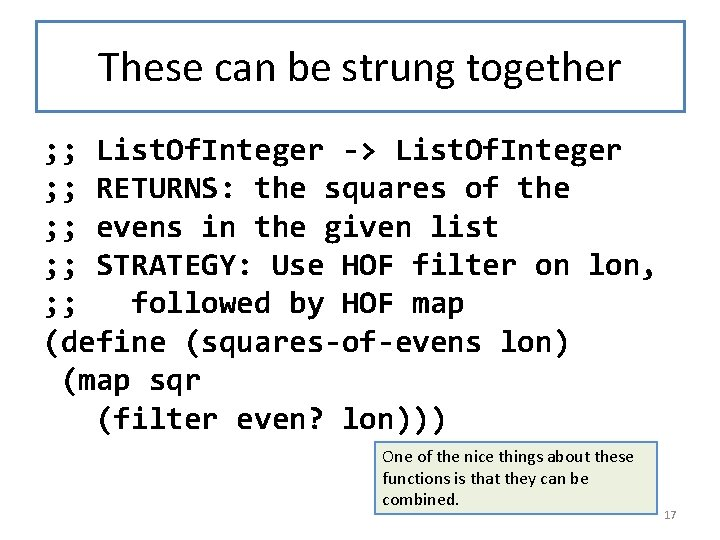These can be strung together ; ; List. Of. Integer -> List. Of. Integer ; ; RETURNS: the squares of the ; ; evens in the given list ; ; STRATEGY: Use HOF filter on lon, ; ; followed by HOF map (define (squares-of-evens lon) (map sqr (filter even? lon))) One of the nice things about these functions is that they can be combined. 17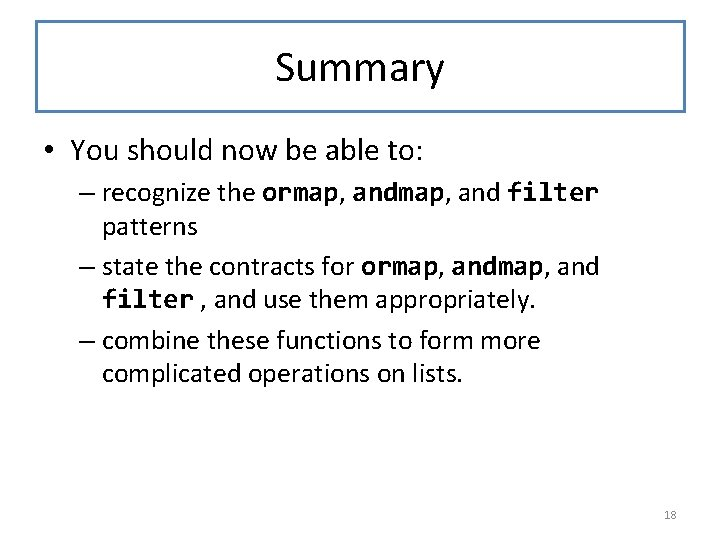Summary • You should now be able to: – recognize the ormap, and filter patterns – state the contracts for ormap, and filter , and use them appropriately. – combine these functions to form more complicated operations on lists. 18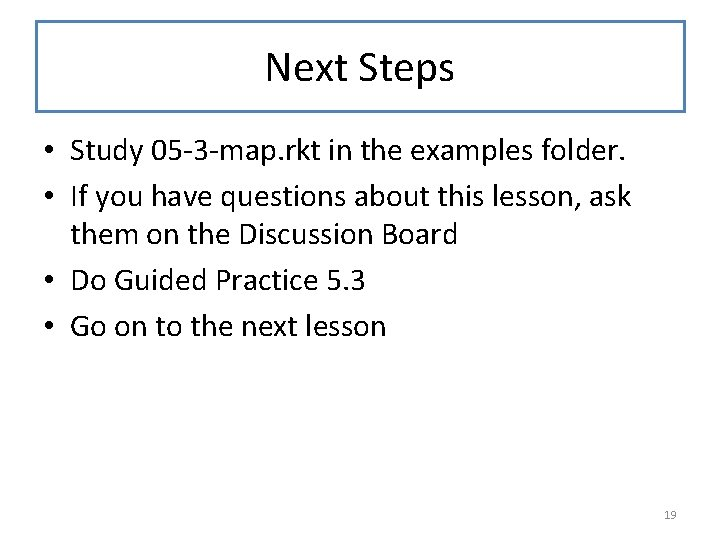Next Steps • Study 05 -3 -map. rkt in the examples folder. • If you have questions about this lesson, ask them on the Discussion Board • Do Guided Practice 5. 3 • Go on to the next lesson 19